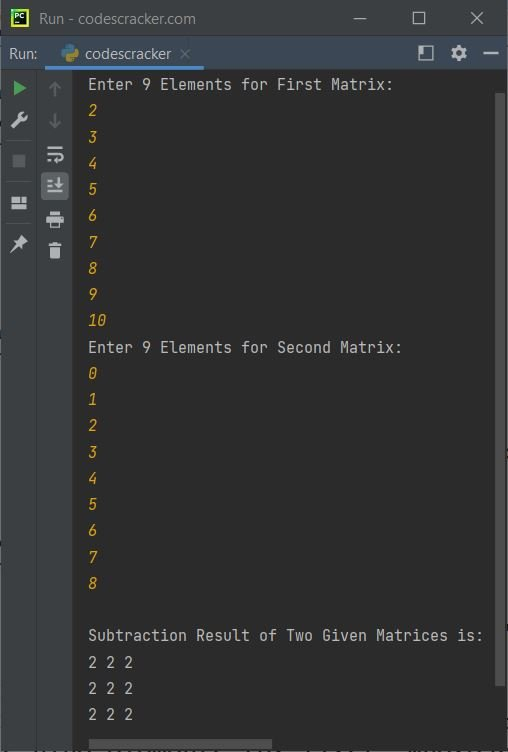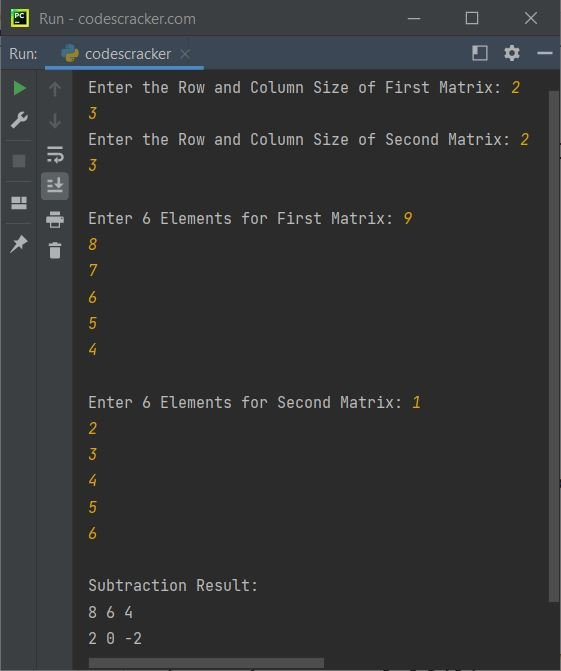# Python Program to Subtract Two Matrices

• Subtract two 3*3 matrices - without user input
• Subtract two 3*3 matrices - with user input
• Subtract two matrices of given order and elements

## Subtract Two Matrices in Python

The question is, write a Python program to perform matrix subtraction. The program given below is its answer:

```matrix1 = [[10, 11, 12],
[13, 14, 15],
[16, 17, 18]]
matrix2 = [[1, 2, 3],
[4, 5, 6],
[7, 8, 9]]
rmatrix = [[0, 0, 0],
[0, 0, 0],
[0, 0, 0]]

for i in range(len(matrix1)):
for j in range(len(matrix1)):
rmatrix[i][j] = matrix1[i][j] - matrix2[i][j]

for r in rmatrix:
print(r)```

The output produced by above Python program is:

```[9, 9, 9]
[9, 9, 9]
[9, 9, 9]```

The subtraction of two matrices in above program can be calculated as:

• matrix1 - matrix2 = 10 - 1 = 9 = rmatrix
• matrix1 - matrix2 = 11 - 2 = 9 = rmatrix
• matrix1 - matrix2 = 12 - 3 = 9 = rmatrix
• matrix1 - matrix2 = 13 - 4 = 9 = rmatrix
• matrix1 - matrix2 = 14 - 5 = 9 = rmatrix
• matrix1 - matrix2 = 15 - 6 = 9 = rmatrix
• matrix1 - matrix2 = 16 - 7 = 9 = rmatrix
• matrix1 - matrix2 = 17 - 8 = 9 = rmatrix
• matrix1 - matrix2 = 18 - 9 = 9 = rmatrix

## Python Subtract Two Given 3*3 Matrices

The question is, write a Python program to subtract two matrices. Elements for both the matrix must be received by user at run-time of the program. Answer to this question, is the program given below:

```matOne = []
print("Enter 9 Elements for First Matrix: ")
for i in range(3):
matOne.append([])
for j in range(3):
num = int(input())
matOne[i].append(num)

matTwo = []
print("Enter 9 Elements for Second Matrix: ")
for i in range(3):
matTwo.append([])
for j in range(3):
num = int(input())
matTwo[i].append(num)

matThree = []
for i in range(3):
matThree.append([])
for j in range(3):
sub = matOne[i][j] - matTwo[i][j]
matThree[i].append(sub)

print("\nSubtraction Result of Two Given Matrices is:")
for i in range(3):
for j in range(3):
print(matThree[i][j], end=" ")
print()```

The snapshot given below shows the sample run of above python program with user input 2, 3, 4, 5, 6, 7, 8, 9, 10 as nine elements for first, whereas 0, 1, 2, 3, 4, 5, 6, 7, 8 as nine elements for second matrix:## Python Subtract Two Given Matrices

This is the last program of this article, created to allow user to define the order and elements for both the matrices.

```print("Enter the Row and Column Size of First Matrix: ", end="")
rowOne = int(input())
colOne = int(input())
print("Enter the Row and Column Size of Second Matrix: ", end="")
rowTwo = int(input())
colTwo = int(input())

if rowOne==rowTwo and colOne==colTwo:
matOne = []
print("\nEnter", rowOne*colOne, "Elements for First Matrix: ", end="")
for i in range(rowOne):
matOne.append([])
for j in range(colOne):
num = int(input())
matOne[i].append(num)

matTwo = []
print("\nEnter", rowTwo*colTwo, "Elements for Second Matrix: ", end="")
for i in range(rowTwo):
matTwo.append([])
for j in range(colTwo):
num = int(input())
matTwo[i].append(num)

matThree = []
for i in range(rowOne):
matThree.append([])
for j in range(colTwo):
sub = matOne[i][j] - matTwo[i][j]
matThree[i].append(sub)

print("\nSubtraction Result:")
for i in range(rowOne):
for j in range(colOne):
print(matThree[i][j], end=" ")
print()
else:
print("\nOrder Mismatched!")```

The sample run of above Python program, is shown in the snapshot given below:#### Same Program in Other Languages

Python Online Test

« Previous Program Next Program »